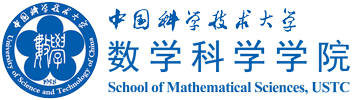Teaching-Liu(Spring 2022)

## Notices

 2.Final exam: Room 5503: 2020.06.14 14:30-16:30. 1. Lecture room 5403: 2(15:55-18:20); 5(14:00-15:35).

## Lectures and excercises

 Lecture 1 Introduction; Manifolds; Tangent spaces; Riemannian metric. Lecture 2 Tensors; Existence of Riemannian metric; Distance function and metric structure. Explanation on paracompactness and the concept of tensors Lecture 3 Looking for shortest curves in Eulidean and Spherical geometry; Energy functional; geodesic equation. Lecture 4 Looking for charts with simple geodesic equations: exponential map. Why do we choose Christoffel symbols to be symmetric? Lecture 5 Local existence and uniqueness of shortest curves; Totally normal neighborhood; Cut point. Lecture 6 Existence of shortest curves between any two points: Completeness. Lecture 7 Hopf-Rinow Theorem; Existence of closed geodesics. Lecture 8 Existence of shortest curves in a given homotopy class; Riemannian covering maps. Lecture 9 Affine connections; Vector fields along a curve; Parallelism. Lecture 10 Covariant derivatives of tensor fields; Affine connections determined by Christoffel symbols. Lecture 11 Levi-Civita connection; First and second variation formulae; Curvature tensor. Lecture 12 Curvature tensor: Integrability condition; Covariant differentiation and Ricci identity. Lecture 13 Local version of Ricci identity; Hessian, divergence and gradient. blackboard note pdf, png Lecture 14 Laplacian; Bianchi identities; Symmetries of Riemannian curvature tensor. blackboard note pdf, png Lecture 15 Sectoional, Ricci and scalar curvatures; Schur Theorem. blackboard note pdf, png Lecture 16 Bochner identity; Second Variation Formula revisited; Synge Theorem. Lecture 17 Bonnet-Myers Theorem; Jacobi field; Length of geodesic circles. Lecture 18 Jacobi field and conjugate points. Lecture 19 Index forms. Lecture 20 Index Lemma; Morse Index Theorem; Cartan-Hadamard Theorem Lecture 21 Uniqueness of simply connected space form: non-positive curvature. Lecture 22 Uniqueness of simply connected space form: positive curvature; Convexity; Cut points revisited. Lecture 23 Continuity of cut locus; Sturm comparison theorem and its geometric translations by Bonnet. Lecture 24 Transport a vector filed along a curve to another space; Morse-Schoenberg comparison theorem; Rauch comparison theorem; Sum of the angles of a geodesic triangle in nonpositively curved manifolds. Lecture 25 Hessian comparison theorem. Lecture 26 Laplacian comparison theorem; volume density and Jacobi fields. Lecture 27 Volume and Riemannian measure; Relating volume to Laplacian. Lecture 28 Bishop-Gromov volume comparison theorem; Cheng's Maximal diameter theorem; Complete Riemannian manifolds with nonnegative Ricci curvature: Yau's volume growth rate estimate; Busemann functions. Lecture 29 Cheeger-Gromoll splitting Theorem (I): The Buseman function is harmonic with parallel gradient field. Lecture 30 Cheeger-Gromoll splitting Theorem (II): Totally geodesic submanifolds and the isometric map. Homework 1 Christoffel symbols and Geodesics.

## Tutorials

 Öś½Ģ Ģļ¬Bź» tian18@mail.ustc.edu.cn »ĘĪÄČš hwr24@mail.ustc.edu.cn